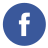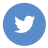### java AES encrypt and decrypt

java

In Java, you can use the Cipher class from the javax.crypto package to perform AES encryption and decryption. Here’s an example of how you can use the Cipher class to encrypt and decrypt a message in Java:

``````import java.security.Key;
import javax.crypto.Cipher;
import javax.crypto.spec.SecretKeySpec;
import java.util.Base64;

public class Main {
public static void main(String[] args) throws Exception {
// Set up the Cipher object

// Create a secret key
byte[] keyBytes = "0123456789012345".getBytes("UTF-8");
Key key = new SecretKeySpec(keyBytes, "AES");

// Encrypt the message
cipher.init(Cipher.ENCRYPT_MODE, key);
byte[] encryptedBytes = cipher.doFinal("Hello, world!".getBytes("UTF-8"));
String encryptedString = Base64.getEncoder().encodeToString(encryptedBytes);
System.out.println(encryptedString);

// Decrypt the message
cipher.init(Cipher.DECRYPT_MODE, key);
byte[] decryptedBytes = cipher.doFinal(Base64.getDecoder().decode(encryptedString));
String decryptedString = new String(decryptedBytes, "UTF-8");
System.out.println(decryptedString);
}
}``````

This code will output the encrypted version of the message “Hello, world!” and then output the decrypted version of the same message.

Note that in this example, the secret key is a simple string that is used as the key for the AES algorithm. In a real application, you should use a more secure key, such as one that is generated using a key derivation function like PBKDF2.